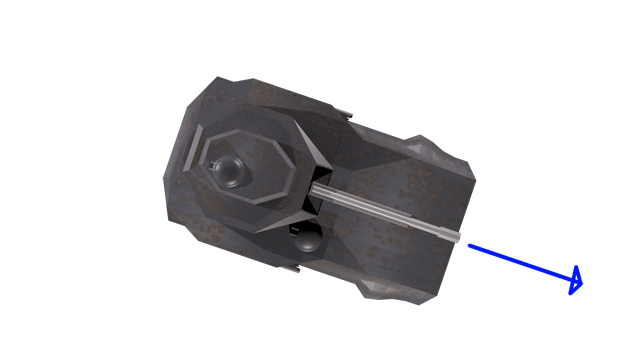DEFCOM1

## Translation and Rotation

 Adding Key Control Time Based Movement Translation Maths

In our last game we simply used the keys to add values to the X and Y coordinates (Position) to move out sprite up, down and across the screen. That is all well and good unless we want to move at a specific angle.To do that we simply need a bit of trigonometry :)

We take the current position and multiply the velocity by the Cos and Sin of the anlgle, it looks something like this.

X += Velocity * Cos(angle)

Y += Velocity * SIn(angle)

So let’s say your tank was currently 100 pixels across and 100 pixels down, traveling at 10 pixels per frame at an angle of 15 degrees

New X = 100 + (10 * Cos(15))

New Y = 100 + (10 * Sin(15))

New X = 109.65

New Y = 102.58

### Adding Key Control and Time based Movement

Go to the Update Function and add the following code. It is practically the same except for one new line, the new elapsed variable. Elapsed is the calculation of how much time has passed since the last time the update function was called.

This will allow us to move our sprite based on the amount of time between frames

```KeyboardState key = Keyboard.GetState();
float elapsed = (float)gameTime.ElapsedGameTime.TotalSeconds;

if (key.IsKeyDown(Keys.Left))
{

}
if (key.IsKeyDown(Keys.Right))
{

}
if (key.IsKeyDown(Keys.Up))
{

}
if (key.IsKeyDown(Keys.Down))
{

}```

### Rotation

Simply update the rotation value when pressing Left and Right

```if (key.IsKeyDown(Keys.Left))
{
rotation -= 1.0f * elapsed;
}
if (key.IsKeyDown(Keys.Right))
{
rotation += 1.0f * elapsed;
}
```

Press F5, you should now be able to rotate the tank using the arrow keys

Translation

Now we have a rotation angle, we can use the formula

X += Velocity * Cos(angle)

Y += Velocity * SIn(angle)

to move our tank the direction it is heading.

Important

Computers will nearly always use ‘Radians’ not ‘Degrees

As I am sure you are aware there are 360 degrees in a circle

Don’t worry too much about this, it simply means if you are trying to move along a heading of 180 degrees, you actually need to go along a heading of approximately 3.142

So if you entered 180 when it expected radians, it would in fact be 565 degrees!!!

Add the following code to your Keyboard Up command, please note that I like to create temporary X and Y variables and the add them to the position.

```if (key.IsKeyDown(Keys.Up))
{
float x, y;

x = (100 * elapsed) * (float)Math.Cos(rotation);
y = (100 * elapsed) * (float)Math.Sin(rotation);

position.X += x;
position.Y += y;
}
```

Can you make your tank reverse?

Completed Listings

```public class Game1 : Microsoft.Xna.Framework.Game
{
GraphicsDeviceManager graphics;
SpriteBatch spriteBatch;

//Global Variables
protected Texture2D SpriteSheet;

protected Vector2 position;
protected float rotation;

public Game1()
{
graphics = new GraphicsDeviceManager(this);
Content.RootDirectory = "Content";
}

protected override void Initialize()
{

position = new Vector2();
rotation = 0;

position.X = 200;
position.Y = 200;

base.Initialize();

}

{
// Create a new SpriteBatch, which can be used to draw textures.
spriteBatch = new SpriteBatch(GraphicsDevice);

}

{
// TODO: Unload any non ContentManager content here
}

protected override void Update(GameTime gameTime)
{
// Allows the game to exit
this.Exit();

KeyboardState key = Keyboard.GetState();
float elapsed = (float)gameTime.ElapsedGameTime.TotalSeconds;

if (key.IsKeyDown(Keys.Left))
{
rotation -= 1.0f * elapsed;
}
if (key.IsKeyDown(Keys.Right))
{
rotation += 1.0f * elapsed;
}
if (key.IsKeyDown(Keys.Up))
{
float x, y;

x = (100 * elapsed) * (float)Math.Cos(rotation);
y = (100 * elapsed) * (float)Math.Sin(rotation);

position.X += x;
position.Y += y;
}

if (key.IsKeyDown(Keys.Down))
{
float x, y;

x = (-100 * elapsed) * (float)Math.Cos(rotation);
y = (-100 * elapsed) * (float)Math.Sin(rotation);

position.X += x;
position.Y += y;
}

base.Update(gameTime);
}

protected override void Draw(GameTime gameTime)
{

GraphicsDevice.Clear(Color.SeaGreen);

spriteBatch.Begin();

spriteBatch.Draw(SpriteSheet, position, new Rectangle(0, 0, 128, 128),
Color.White, rotation, new Vector2(64, 64), 1.0f, SpriteEffects.None, 1);

spriteBatch.End();

base.Draw(gameTime);
}
```